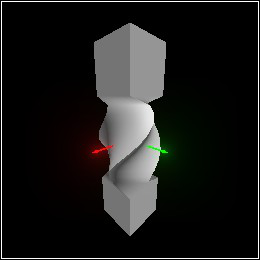### 7Deformation and Tessellation

TODO: exposition about deformation and tessellation and Jacobian

#### 7.1Basic Deformation Data Types

 type
 predicate
The type and predicate for three-dimensional, almost-everywhere continuously differentiable functions. This includes Affine (and thus Linear) as a subtype.

 procedure(smooth f) → Smooth f : (-> Pos Pos) (smooth f j) → Smooth f : (-> Pos Pos) j : (-> Pos Linear)
Creates a deformation function. If only f is given, it is numerically differentiated to construct the Jacobian function. Otherwise, j is used.

Warning: If f is numerically differentiated, it may be evaluated outside of its intended domain.

 procedure(smooth-function t) → (-> Pos Pos) t : Smooth
 procedure t : Smooth
Return the function represented by t and its Jacobian matrix of partial derivatives.

When t is Linear or Affine, (smooth-function t) is equivalent to (λ (v) (transform-pos v t)).

When t is Linear, (smooth-jacobian t) is equivalent to (λ (_) t).

When t is Affine, (smooth-jacobian t) is equivalent to
 (match-let ([(affine dx dy dz v)  t]) (λ (_) (linear dx dy dz)))

 value
The identity deformation. Equivalent to identity-linear and identity-affine.

 procedure(smooth-compose t ...) → Smooth t : Smooth
Composes any number of deformations. Applying the result applies each t once, in reverse order (just like compose).

Example:
 > (combine (deform (tessellate (rectangle (pos 0 0 2) (dir 1/8 1/4 2)) #:segments 48) (smooth-compose (move-x (/ -2 pi)) (bend 360 (interval 0 4)) (twist 45) (rotate-z 45))) (basis 'camera (point-at (pos 1/2 1 1/2) (pos 1/8 0 1/8))))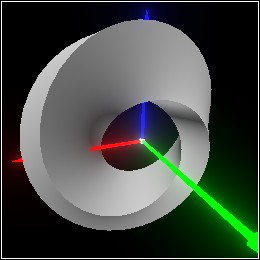procedure t : Smooth v : Pos
Returns the best linear approximation of t at point v.

If t is Linear or Affine, this simply returns t. Otherwise, (smooth-approximate t v) is equivalent to
 (match-let ([(linear dx dy dz)  ((smooth-jacobian f) v)]) (define v0 ((smooth-function f) v)) (affine dx dy dz (pos+ origin (pos- v0 v))))

 procedure t : Smooth v : Pos
Returns #t when inverting ((smooth-jacobian t) v) would raise an error. See linear-inverse and linear-singular?.

 procedure t : Smooth v : Pos
Returns #t when t preserves orientation, or handedness, at v. See linear-consistent?.

#### 7.2Tessellation

procedure

 (tessellate pict [ #:segments segments #:max-edge max-edge #:max-angle max-angle]) → Pict3D
pict : Pict3D
segments : Integer = (current-tessellate-segments)
max-edge : (U #f Real) = (current-tessellate-max-edge)
max-angle : Real = (current-tessellate-max-angle)
Approximates pict with triangles so deformations can be applied to their vertices.

Each kind of shape has its own tessellation algorithm, which follows the these general guidelines:
• Edges that approximate part of an arc represent no more than max-angle degrees of the arc.

• Other edges are shorter than max-edge.

When max-edge is #f (the default), it’s computed as (/ l segments), where l is the length of the longest axis of pict’s bounding rectangle.

Examples:
 > (combine (tessellate (rectangle origin (dir 1/4 1/4 1)) #:segments 4) (basis 'camera (point-at (pos 0.75 0.75 1.25) origin)))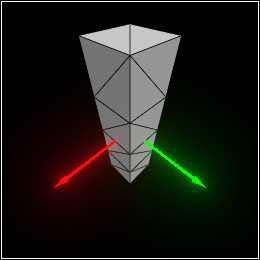> (tessellate (sphere origin 1/2) #:max-angle (/ 90 4))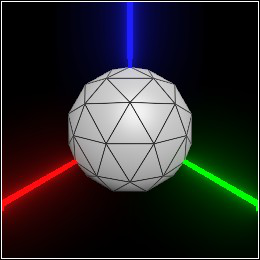> (tessellate (cylinder origin 1/2 #:arc (arc 90 45)) #:max-angle 45 #:max-edge 1/4)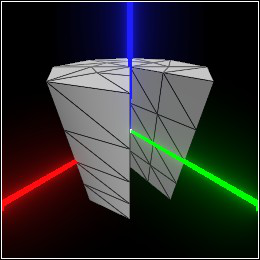> (tessellate (cone origin 1/2) #:max-angle 30 #:segments 32)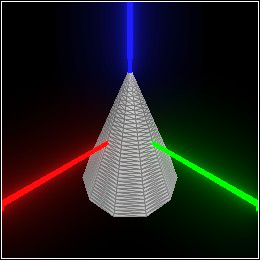procedure

 (adaptive-tessellate pict [ t #:segments segments #:max-edge max-edge #:max-angle max-angle #:max-iters max-iters]) → Pict3D
pict : Pict3D
t : Smooth = identity-smooth
max-edge : (U #f Real) = (current-adaptive-max-edge)
Tries to tessellate pict in a way that reduces visual artifacts when deformed by t. This function is available for completeness; usually adaptive-deform is more appropriate.

Examples:
 > (define c (basis 'camera (point-at (pos 2.5 1.5 1.0) (dir -0.75 -0.5 -0.25))))
> (define t (bend 90 (interval -1/2 1/2)))
> (define p (adaptive-tessellate (cylinder origin (dir 1/2 1/2 2)) t))
> (combine c p)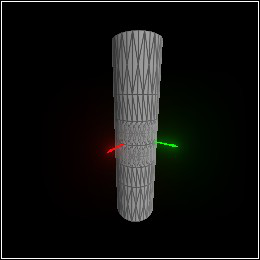> (combine c (deform p t))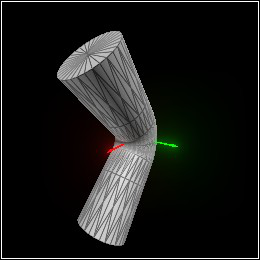Except for max-iters, the keyword arguments have similar meaning to those of tessellate. However, adaptive-tessellate
1. Requests an initial tessellation of each shape with max-angle = 90 and max-edge = +inf.0.

2. For up to max-iters iterations, splits triangle edges that, when transformed, would be too long or represent too many degrees of an arc, and moves the new vertex onto the shape’s surface.

3. For up to 3 iterations, splits triangle edges that change orientation when transformed, using flbracketed-root to solve for locations at which t’s Jacobian determinant is zero.

This is a lot of extra work, which usually can’t be done at interactive speeds. On the other hand, adaptive-tessellate sometimes uses fewer triangles (this is still a work in progress), and can deal better with deformations with discontinuities and local noninvertibility.

Examples:
 > (define t (smooth (λ (v) (match-define (pos x y z) v) (pos x y (* x z)))))
> (deform (tessellate (ellipsoid (pos -1/3 0 0) (dir 1 1/2 1/2))) t)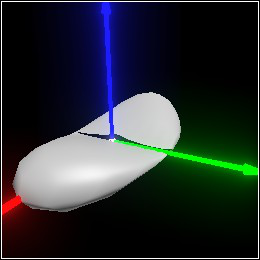> (adaptive-deform (ellipsoid (pos -1/3 0 0) (dir 1 1/2 1/2)) t)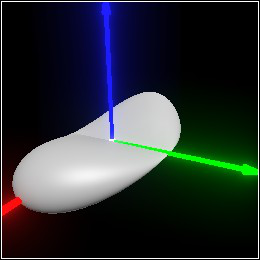procedure

(current-tessellate-segments segments)  Void
segments : Integer
 = 12

procedure

(current-tessellate-max-edge max-edge)  Void
max-edge : (U #f Real)
 = #f

procedure

(current-tessellate-max-angle max-angle)  Void
max-angle : Real
 = 15
Default keyword argument values for tessellate.

procedure

segments : Integer
 = 0

procedure

max-edge : (U #f Real)
 = #f

procedure

max-angle : Real
 = 15

procedure

max-iters : Integer
 = 5

#### 7.3Deformation Constructors and Combiners

 procedure(deform-pos v t) → Pos v : Pos t : Smooth
 procedure(deform-dir v dv t) → Dir v : Pos dv : Dir t : Smooth
 procedure(deform-norm v dv t) → (U #f Dir) v : Pos dv : Dir t : Smooth
Apply deformation t to a position, direction or normal.

These are analogous to transform-pos, transform-dir and transform-norm, respectively. (In fact, when t is Affine, they simply apply their affine counterparts.) The main difference is that deform-dir and deform-norm require a position argument to apply the Jacobian to.

 procedure(deform-affine t0 t) → (U #f Affine) t0 : Affine t : Smooth
Applies deformation t to an affine transformation.

If t is Affine, this is equivalent to (affine-compose t t0). Otherwise, it’s equivalent to
 (match-let ([(affine dx dy dz v)  t0]) (affine (deform-dir v dx t) (deform-dir v dy t) (deform-dir v dz t) (deform-pos v t)))

Group transformations, and untessellated solid objects such as spheres, are deformed using deform-affine.

 procedure(deform pict t) → Pict3D pict : Pict3D t : Smooth
Deforms pict by applying t to its positions, normals and affine transformations.

procedure

 (adaptive-deform pict t [ #:segments segments #:max-edge max-edge #:max-angle max-angle #:max-iters max-iters]) → Pict3D
pict : Pict3D
t : Smooth
max-edge : (U #f Real) = (current-adaptive-max-edge)
Tries to tessellate pict in a way that reduces visual artifacts when deformed by t, and then deforms the tessellation by t. See adaptive-tessellate for discussion and examples.

 procedure(local-deform t local-t) → Smooth t : Smooth local-t : Affine (local-deform pict t local-t) → Pict3D pict : Pict3D t : Smooth local-t : Affine
Applies t to pict in the local coordinate space defined by local-t, or returns a Smooth function that does so. Analogous to local-transform.

The two-argument version is equivalent to (smooth-compose local-t t (affine-inverse local-t)). In English, undo local-t, do t, then redo local-t.

 procedure(twist pict angle) → Pict3D pict : Pict3D angle : Real
Twists pict around the z axis, angle degrees per unit height.

Example:
 > (twist (tessellate (pipe origin (dir 1 1/2 1))) 90)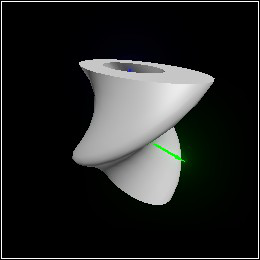In the above example, the pipe is twisted 180 degrees: 90 degrees for one unit below the origin, and 90 degrees for one unit above the origin.

 procedure(displace pict f) → Pict3D pict : Pict3D f : (-> Flonum Flonum Real)
Adds (f x y) to every point’s z coordinate in pict.

Example:
 > (displace (tessellate (rectangle origin (dir 2/3 2/3 1/4))) (λ (x y) (- (sqr x) (sqr y))))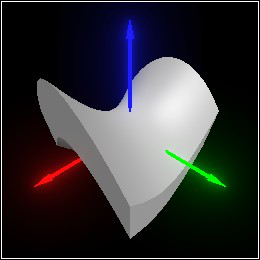procedure(bend pict angle) → Pict3D pict : Pict3D angle : Real (bend pict angle zrange) → Pict3D pict : Pict3D angle : Real zrange : Interval
Bends pict by bending an interval of the z axis angle degrees counterclockwise around the y axis.

If zrange is given, only that interval on the z axis is bent; otherwise, the entire z extent of pict is used. The bent interval always retains its length.

Example:
 > (combine (bend (tessellate (ellipsoid origin (dir 1/8 1/16 1))) 270) (with-color (rgba "orange") (bend (tessellate (rectangle origin (dir 1/8 1/4 1))) -180)) (with-color (rgba "lightgreen" 0.5) (bend (tessellate (cone (pos 0 0 1) (dir 1/4 1/8 1))) 90 (interval 1/2 2))) (with-color (rgba "crimson" 0.5) (bend (tessellate (cylinder (pos 0 0 -1) (dir 1/4 1/8 1))) -45 (interval -1/2 -2))) (basis 'camera (point-at (pos 0.8 1.6 0.5) (pos 0 0 1/4))))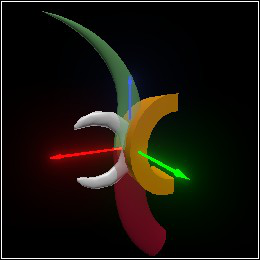procedure(bend angle zrange) → Smooth angle : Real zrange : Interval
 procedure(twist angle) → Smooth angle : Real
 procedure(displace f) → Smooth f : (-> Flonum Flonum Real)
Deformation-returning versions of the above.

 procedure(smooth-between t1 t2 α) → Smooth t1 : Smooth t2 : Smooth α : (U Real (-> Pos Real))
Returns an average of t1 and t2, weighted by α. When α is a function, the weight at each point v is (α v).

One use for smooth-between is to apply a deformation to a small pocket of space, by blending between that deformation and identity-smooth.

Examples:
> (require plot)
> (define (f1 z) (exp (- (sqr z))))
> (define (f2 z) (if (> (sqr z) 1) 0 (sqrt (- 1 (sqr z)))))
 > (plot (list (function f1 #:label "f1") (function f2 #:color 2 #:label "f2")) #:x-min -2 #:x-max 2 #:y-min 0 #:y-max 1 #:x-label "z" #:y-label "α")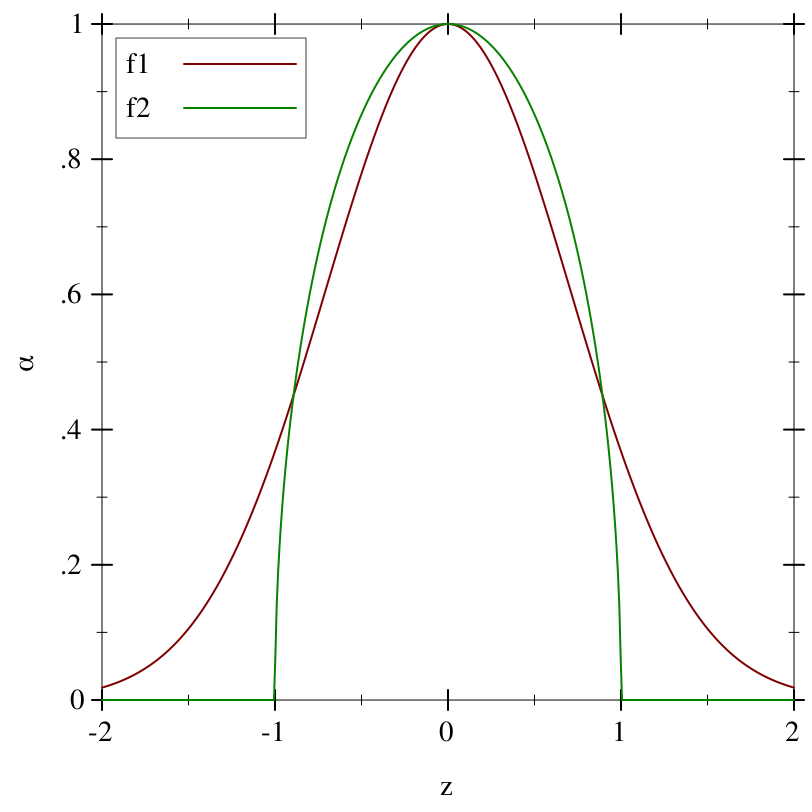> (define (α1 v) (f1 (pos-z v)))
> (define (α2 v) (f2 (pos-z v)))
> (define p (tessellate (rectangle origin (dir 1/2 1/2 2)) #:segments 24))
> (define c (basis 'camera (point-at (pos 2 2 1) (pos 0 0 1/4))))
> (combine c (deform p (twist 90)))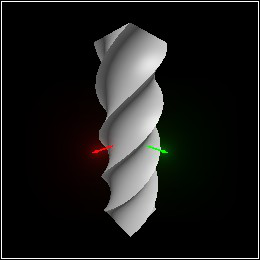> (combine c (deform p (smooth-between identity-smooth (twist 90) α1)))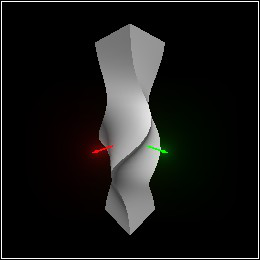> (combine c (deform p (smooth-between identity-smooth (twist 90) α2)))